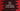# Union and intersection of two HashSet in Dart## How to find the union and intersection of two HashSet in Dart:

In this post, we will discuss on union and intersection of two HashSets. If SetA and SetB are two sets, the union of these two sets will include all the elements which are either in one of these sets. Similarly, the intersection will hold only the common elements of these sets.

The union of SetA and SetB is denoted by SetA ∪ SetB and the intersection is denoted by SetA ∩ SetB.

To find the union and intersection of two HashSets, this class provides two methods. We can use these methods without writing our own utility functions.

### How to find the union of two HashSet:

To find the union of two HashSet, we can use the union method. This method is defined as:

``union(Set<E> o) → Set<E>``

It takes another HashSet o as the parameter and returns the union hashSet of the two sets.

For example,

``````import 'dart:collection';

void main() {
final firstSet = HashSet.of([1, 2, 3, 4]);
final secondSet = HashSet.of([5, 6, 7, 8]);

final unionSet = firstSet.union(secondSet);

print('Union HashSet: \${unionSet}');
}``````

In this example, we are using the union method to find the union of the sets firstSet and secondSet. If you run this program, it will print:

``Union HashSet: {1, 2, 3, 4, 5, 6, 7, 8}``

The content of both of these HashSet shouldn’t be different. Otherwise, it will throw an error. For example,

``````import 'dart:collection';

void main() {
final firstSet = HashSet.of([1, 2, 3, 4]);
final secondSet = HashSet.of(['a', 'b']);

final unionSet = firstSet.union(secondSet);

print('Union HashSet: \${unionSet}');
}``````

This program will throw one error because the data types of firstSet and secondSet are different.

### How to find the intersection of two HashSet:

Similar to union, we have another method to find the intersection of two HashSets. This method is:

``intersection(Set<Object?> o) → Set<E>``

It takes another HashSet as its parameter and returns one new set holding the intersection result. Let’s try it with an example:

``````import 'dart:collection';

void main() {
final firstSet = HashSet.of([1, 2, 3, 4]);
final secondSet = HashSet.of([5, 6, 7, 3, 9, 4]);

final intersectionSet = firstSet.intersection(secondSet);

print('Intersection HashSet: \${intersectionSet}');
}``````

It will print the intersection of firstSet and secondSet:

``Intersection HashSet: {3, 4}``

If no common element is found in the sets, it will print an empty set:

``````import 'dart:collection';

void main() {
final firstSet = HashSet.of([1, 2, 3, 4]);
final secondSet = HashSet.of([5, 6, 7, 8, 9]);

final intersectionSet = firstSet.intersection(secondSet);

print('Intersection HashSet: \${intersectionSet}');
}``````

It will print:

``Intersection HashSet: {}``

### Chain operation of union and intersection:

Since both union and intersection methods return a new set, we can use these in a chain. For example,

``````import 'dart:collection';

void main() {
final firstSet = HashSet.of([1, 2, 3, 4]);
final secondSet = HashSet.of([5, 6, 7, 3, 9]);
final thirdSet = HashSet.of([8, 10, 3]);

final intersectionSet =
firstSet.intersection(secondSet).intersection(thirdSet);
final unionSet = firstSet.union(secondSet).union(thirdSet);

print('Intersection HashSet: \${intersectionSet}');
print('Union HashSet: \${unionSet}');
}``````

This program will print the intersection and union of the three sets firstSet, secondSet and thirdSet.

``````Intersection HashSet: {3}
Union HashSet: {1, 2, 3, 4, 5, 6, 7, 8, 9, 10}``````